Next: 2.4 Summary and outlook Up: 2. The Kondo Model Previous: 2.2.3 Calculation of physical

# 2.3 Results

One quantity that can be calculated analytically is the low-energy limit of the spin structure factor,(2.20)

For a vanishing local magnetic fieldis just the spin relaxation rate accessible in e.g. spin resonance experiments. With the result for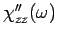from (2.19) we obtain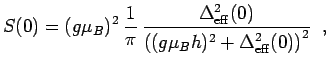(2.21)

which leads to the curve shown in Fig. 2.2. Eq. (2.21) is of particular importance because it explicitly demonstrates universality,, and allows to directly fit e.g. experimental data from ESR or NMR experiments and extract the Kondo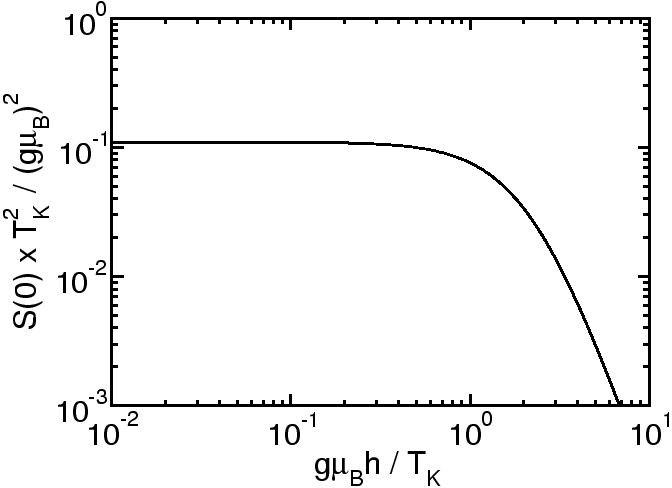temperature. Note furthermore that the result (2.21) is not only valid in the Kondo limit, but also holds at the Toulouse point of the anisotropic Kondo model and everywhere in between. Since it does not depend on the details ofit will also be true for general band structuresin (2.1) and thus is eventually the result forin DMFT calculations.

The full frequency dependenthas to be calculated numerically using the form of the effective hybridization function in Fig. 2.1. The results for three values of the external field,,andare displayed in Fig. 2.3. These correlation functions provide a good example for the usefulness of our mapping to the effective RLM since one can directly interpret the structures and their frequency and field dependencies in terms of analytical formulas derived for the RLM. E.g. the high-frequency behavior offollows directly from equ. (2.19) and the behavior of the effective hybridization functionat large energies (which is linear with logarithmic corrections, see Fig. 2.1):decays likewith logarithmic corrections, in agreement with (expensive) numerical results (23).

For the dependence of the dynamical susceptibility on the local magnetic field one makes use of the fact that the local magnetic field corresponds to the on-site energy in the effective RML. Therefore it is obvious that the observed shift of the resonance peak inis due to the shifted center of the resonant level. Furthermore, the depletion of the maximum value is related to the decreasing occupation of the resonant level, which corresponds directly to the increasing local magnetization in the SIKM. At the same time, one observes a decrease of the total spectral weight in, which can be accounted for by a transfer to a finite expectation value ofin the SIKM. There is, however, also a non-trivial effect, namely the increasing broadening of the resonance peak with increasing magnetic field. For a RLM with a constantsuch a behavior does not occur; it is entirely related to the fact that with increasing magnetic field the system starts to notice the energy dependence of the effective hybridization.

The quantity not yet fixed in our calculation is, or more precisely the proportionality constant in. This can most conveniently be done by using Wilson's definition of the Kondo temperature(3)(2.22)

where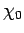is the static magnetic susceptibility andthe Wilson number.can be obtained from the imaginary part of the dynamic susceptibility (2.19) via(2.23)

and must in general be evaluated numerically. At the Toulouse point one can, however, give an analytic answer since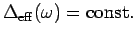and thus(2.24)

Therefore at the Toulouse point the Korringa-Shiba relation(24)(2.25)

is independent of the local magnetic field(2.26)

In the following we will discuss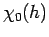and the Korringa-Shiba relation for the Kondo limit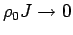. The quantityis particularly convenient for a comparison with NRG results.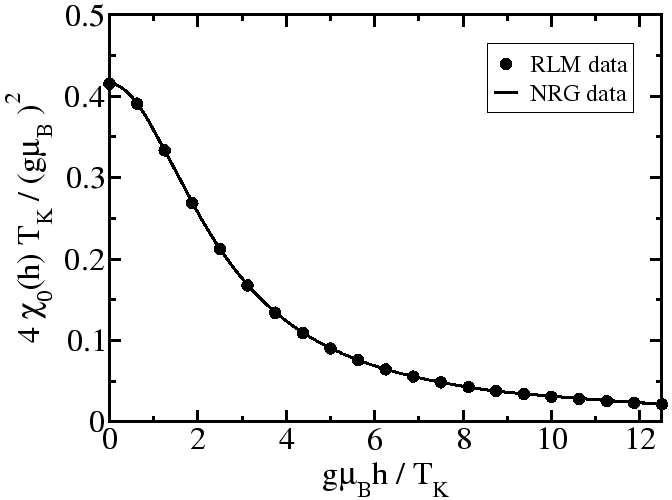In Fig. 3.3 the circles represent the values ofcalculated via (2.23) with the effective hybridization function from Fig. 2.1, and the full line represents the result of an NRG calculation. We observe excellent agreement for all values of the local magnetic field: notice that the curves agree without fit parameters. This example clearly demonstrates that the nontrivial form of the effective hybridization in Fig. 2.1 encodes the many-particle physics of the SIKM in a trivial noninteracting effective model.

The result in Fig. 3.3 can readily be combined with relations (2.17) and (2.21) to obtain the Wilson ratio(5)(2.27)

Our results for the Wilson ratio and the Korringa-Shiba relation obtained within the effective RLM are collected in Fig. 2.5. For the Wilson ratio we would actually have to calculate the quantityand not.(5) However, for the case of small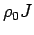considered here, both quantities are equivalent.(25) One observes that bothand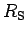are independent of the local magnetic field up to approximately, and then start todecrease (Shiba ratio), respectively increase (Wilson ratio). The exact Bethe ansatz solution (4) givesindependent of the magnetic field strength (see also Ref. (12)), and local Fermi liquid theory yieldsfor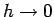.(3) Our limiting values asmiss these exact results by approximately 5%. Notice that the term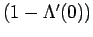in (2.17) is very important to obtain this correct value for. Remarkably, our simple noninteracting effective model therefore correctly describes the Wilson ratio in the Kondo limit (for not too large magnetic fields), which is a hallmark of strong-coupling Kondo physics.

Let us finally analyze the accuracy of our effective model. Since Fig. 3.3 demonstrates that integral quantities likeare obtained with very good accuracy for all magnetic fields, one can infer from Fig. 2.5 that quantities depending on low-energy details in frequency space likeandare more susceptible to our approximations for increasing magnetic fields. This suggests that for such low-energy quantities our effective model can be employed with very good accuracy (5% error) for magnetic fields below, and with good accuracy (20% error) still up to approximately.Next: 2.4 Summary and outlook Up: 2. The Kondo Model Previous: 2.2.3 Calculation of physical
© Cyrill Slezak